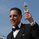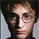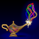4702 lượt xem
This indicator plots the moving average described in the January, 1998 issue
of S&C, p.57, "Smoothing Techniques for More Accurate Signals", by Tim Tillson.
This indicator plots T3 moving average presented in Figure 4 in the article.
T3 indicator is a moving average which is calculated according to formula:
T3(n) = GD ( GD ( GD (n))),
where GD - generalized DEMA ( Double EMA ) and calculating according to this:
GD (n,v) = EMA (n) * (1+v)-EMA( EMA (n)) * v,
where "v" is volume factor, which determines how hot the moving average’s response
to linear trends will be. The author advises to use v=0.7.
When v = 0, GD = EMA , and when v = 1, GD = DEMA . In between, GD is a less aggressive
version of DEMA . By using a value for v less than1, trader cure the multiple DEMA
overshoot problem but at the cost of accepting some additional phase delay.
In filter theory terminology, T3 is a six-pole nonlinear Kalman filter. Kalman
filters are ones that use the error — in this case, (time series - EMA (n)) —
to correct themselves. In the realm of technical analysis , these are called adaptive
moving averages; they track the time series more aggres-sively when it is making large
moves. Tim Tillson is a software project manager at Hewlett-Packard, with degrees in
mathematics and computer science. He has privately traded options and equities for 15 years.
```////////////////////////////////////////////////////////////
//  Copyright by HPotter v1.0 21/05/2014
// This indicator plots the moving average described in the January, 1998 issue
// of S&C, p.57, "Smoothing Techniques for More Accurate Signals", by Tim Tillson.
// This indicator plots T3 moving average presented in Figure 4 in the article.
// T3 indicator is a moving average which is calculated according to formula:
//     T3(n) = GD(GD(GD(n))),
// where GD - generalized DEMA (Double EMA) and calculating according to this:
//     GD(n,v) = EMA(n) * (1+v)-EMA(EMA(n)) * v,
// where "v" is volume factor, which determines how hot the moving average’s response
// to linear trends will be. The author advises to use v=0.7.
// When v = 0, GD = EMA, and when v = 1, GD = DEMA. In between, GD is a less aggressive
// version of DEMA. By using a value for v less than1, trader cure the multiple DEMA
// overshoot problem but at the cost of accepting some additional phase delay.
// In filter theory terminology, T3 is a six-pole nonlinear Kalman filter. Kalman
// filters are ones that use the error — in this case, (time series - EMA(n)) —
// to correct themselves. In the realm of technical analysis, these are called adaptive
// moving averages; they track the time series more aggres-sively when it is making large
// moves. Tim Tillson is a software project manager at Hewlett-Packard, with degrees in
// mathematics and computer science. He has privately traded options and equities for 15 years.
////////////////////////////////////////////////////////////
study(title="T3 Averages", shorttitle="T3", overlay = true)
Length = input(5, minval=1)
xPrice = close
xe1 = ema(xPrice, Length)
xe2 = ema(xe1, Length)
xe3 = ema(xe2, Length)
xe4 = ema(xe3, Length)
xe5 = ema(xe4, Length)
xe6 = ema(xe5, Length)
b = 0.7
c1 = -b*b*b
c2 = 3*b*b+3*b*b*b
c3 = -6*b*b-3*b-3*b*b*b
c4 = 1+3*b+b*b*b+3*b*b
nT3Average = c1 * xe6 + c2 * xe5 + c3 * xe4 + c4 * xe3
plot(nT3Average, color=blue, title="T3")
```

## Bình luậnHi Harry! Great Work!!!

I found this one looking for a DEMA with a setting so that it can be applied to high-low-open-close prices. Seems there's none in Tradingview.... Do you think you could help me?

Phản hồiDiegoKatzman
Hi Diego,
If I understand you correctly, you can change a line "xPrice = close" on the "xPrice = ohlc4"
Phản hồiHPotter
Thanks! I meant getting just the adjustable DEMA in a separate indicator :D

Do you think you could create one from your script?
Phản hồiHi HP: I just read an article in S&C 2010 where a Kalman filter was utilized to predict or forecast future price trends. Could this indicator be extended to accomplish the same goal?
You can find the article here: http://edmond.mires.co/GES816/22-Predicting%20Market%20Data%20Using%20The%20Kalman%20Filter(1).pdf
Phản hồiStockSwinger
How do you plan on using the Kalman? Ive tried using it for quite some time in different manners. In theory it sounds great. But in my experience, I found it difficult to implement in a reliable and efficient way.
Phản hồicooney_s
HI CD71:

From what I took away from the article the formula can be utilized to project forward what the next several day price actions will be. If you can do that with even a 60% chance of being correct it would be a dramatic improvement of a 50/50 coin flip. HP was utilizing the Kalman code to smooth out a moving Avg, not to forecast which is my intent. I fully realize that forecasting is like predicting the weather with magic, but I got the time to invest to see if there might be some magic in this formula. Ehlers Center of Gravity come pretty close.
Phản hồiThis is a great indicator. Can we please request an Oscillator version. I would like to overlap several T3 averages with different volume factors. Much cleaner to read the momentum within an oscillator. Thanks! Great work.
Phản hồicooney_s
"Can we please request an Oscillator version." Do you have a formula? I can try make it.
Phản hồi